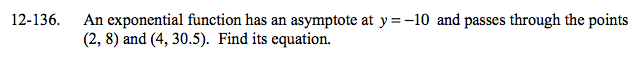### Home > A2C > Chapter 12 > Lesson 12.3.1 > Problem12-136

12-136.

An exponential function has an asymptote at y = −10 and passes through the points (2, 8) and (4, 30.5). Find its equation. Homework Help ✎Use y = abx + k

What do you know is the value of k?

Use the given points to find a and b.